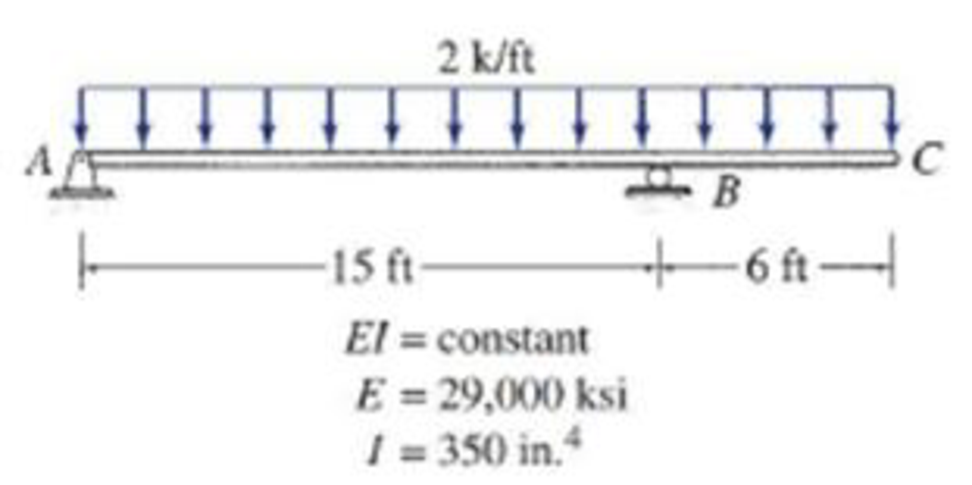# 6.23 through 6.30 Determine the maximum deflection for the beam shown by the moment-area method. FIG. P6.29, P6.55

#### Solutions

Chapter
Section
Chapter 6, Problem 29P
Textbook Problem
669 views

## 6.23 through 6.30 Determine the maximum deflection for the beam shown by the moment-area method.FIG. P6.29, P6.55

To determine

Find the maximum deflection Δmax for the given beam by using moment-area method.

### Explanation of Solution

Given information:

The Young’s modulus (E) is 29,000 ksi.

The moment of inertia (I) is 350in.4.

Calculation:

Consider rigidity modulus (EI) of the beam is constant.

To draw the M/EI the given beam can be divided into two segments such as AB and AC.

Consider span AB:

Show the free body diagram for span AB as in Figure (1).

Determine the support reaction at A;

MB=0RA×15(2×15×152)=0RA=22515RA=15kips

Determine the support reaction at B;

V=0RA+RB(2×15)=0RB=3015RB=15kips

Show the reactions of the beam as in Figure (2).

Determine the bending moment at A using the relation;

MA=15×15(2×15×152)=225225=0

Determine the bending moment at centre of the beam AB;

Mcenter=15×7.5(2×7.5×7.52)=112.556.25=56.25kips-ft

Determine the bending moment at B;

MB=15×15+(2×15×152)=225+225=0

Consider span AC:

The uniformly distributed load is acting in span BC.

Show the free body diagram of the portion BC as in Figure (3).

Determine the support reaction at B;

MA=0RB×15+(2×21×212)=0RB=44115RB=29.4kips()

Determine the support reaction at A;

V=0RA+RB(2×21)=0RA=4229.4RA=12.6kips()

Show the reactions of the beam as in Figure (4).

Determine the bending moment at A;

MA=29.4×15(2×21×212)=405405=0

Determine the bending moment at B;

MB=12.6×15(2×15×152)=189225=36kips-ft

Determine the bending moment at C;

MC=12.6×21(2×21×212)+(29.4×6)=264.6441+176.4=0

Show the M/EI diagram of the given beam as in Figure (5).

Show the elastic curve of the given beam as in Figure (6).

Determine the between point B and A using the relation;

ΔBA=[MEI(Areaofparabola)+MEI(Areaoftriangle)]

Substitute 56.25EI for MEI, (23×15×(152)) for area of parabola, (36EI) for MEI, and (12×15×(13×15)) for area of triangle.

ΔBA=[56.25EI(23×15×(152))+(36EI)(12×15×(13×15))]=1EI(4,218.751,350)=2,868.75kips-ft3EI

Determine the slope at A using the relation;

θA=ΔBAL

Substitute 2,868.75kips-ft3EI for ΔBA and 15 ft for L.

θA=2,868

### Still sussing out bartleby?

Check out a sample textbook solution.

See a sample solution

#### The Solution to Your Study Problems

Bartleby provides explanations to thousands of textbook problems written by our experts, many with advanced degrees!

Get Started

Find more solutions based on key concepts
For problems 15.33 through 15.40, go through each line of MATLAB code and show the result, or indicate if an er...

Engineering Fundamentals: An Introduction to Engineering (MindTap Course List)

Briefly describe an alloy.

Precision Machining Technology (MindTap Course List)

When is a table in 3NF?

Database Systems: Design, Implementation, & Management

Type B engine misfires are excessive if the misfiring exceeds to percent in a rpm period.

Automotive Technology: A Systems Approach (MindTap Course List)

What formula can a project manager use to estimate task duration? Provide an example.

Systems Analysis and Design (Shelly Cashman Series) (MindTap Course List)

List two leading manufacturers of personal computer processor chips. List considerations when choosing a proces...

Enhanced Discovering Computers 2017 (Shelly Cashman Series) (MindTap Course List)

If your motherboard supports ECC DDR3 memory, can you substitute non-ECC DDR3 memory?

A+ Guide to Hardware (Standalone Book) (MindTap Course List)

What is hot work?

Welding: Principles and Applications (MindTap Course List)